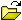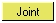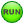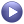# MV-1080: Create an Analysis Using MDL

In this tutorial, you will learn how to create an analysis definition and instantiate it in an MDL file.

An analysis is a collection of loads, motions, output requests, and entities (bodies, joints, and so on) describing a particular event applied to a model. For example, an analysis to determine the kinematics of a four-bar mechanism can be described in one analysis, while another analysis can be used to study a dynamic behavior. In both cases, while the overall model is the same, the analysis container may contain different entities that form the event. The kinematic analysis can contain motion and related outputs, while the dynamic analysis may contain forces and its corresponding outputs.
An analysis definition is similar to a system definition in syntax and usage except for these key differences:
• Analysis definitions use *DefineAnalysis(), while system definitions use *DefineSystem().
• Analysis can be instantiated under the top level Model only.
• Only one analysis can be active in the model at a given instance.
An analysis definition block begins with *DefineAnalysis() and ends with *EndDefine(). All entities defined within this block are considered to be part of the analysis definition. The syntax of *DefineAnalysis() is shown below:
*DefineAnalysis(ana_def_name, arg_1,arg_2, ..., arg_n)
• ana_def_name The variable name of the analysis definition which will be used while instantiating the analysis.
• arg_1,arg_2...arg_n A list of arguments passed to the analysis definition as attachments.
Table 1 illustrates an analysis definition and its subsequent instantiation within an MDL file. Two files, an analysis definition file and the model file, work together when instantiating a particular analysis under study. Some of the terms in the example are in bold to highlight a few key relationships between the files.
Table 1.
Reference Numbers System Instantiation with Definition
1
// Model : Body.mdl
*BeginMDL(base_model, "Base Model")
2
//Instantiate the analysis definition ana_def
*Analysis(ana1, "Analysis 1", ana_def, j_rev)
3
//Begin Analysis Defintion Block
*DefineAnalysis(ana_def,j_joint_att)
4
//Declare a joint attachment to the analysis
*Attachment(j_joint_att, "Joint Attachment", Joint,
"Add an joint external to this analysis to apply
motion/force")
5
//Entities within the analysis
*Point(p_1, "Point in the analysis")
*Body(b_1, "Body in the analysis")
6
//Define a joint with the body b_sys and the body
attachment b_att
*Motion(mot, "Joint Motion", JOINT, j_joint_att,
ROT)
7
*EndDefine() //End Definition Block
8
*EndMDL()
Table 2 details the relationships between the analysis definition and its instantiation in the MDL Model file.
Table 2.
Variable Relationship
j_joint_att The varname of the attachment, declared in the *Attachment() statement (line 4) in the analysis definition file, appears as an argument in the *DefineAnalysis() statement (line 3). A motion is applied on this joint using the *Motion() statement (line 6).
ana_def The varname of the analysis definition is specified in the *DefineAnalysis() statement (line 3). The analysis definition is used by ana1 in the *Analysis() statement (line 2).

## Create the Analysis Definition File

In this step you will create the analysis definition file.

An experimental technique for estimating the natural frequencies of structures is to measure the response to an impulsive force or torque, then look at the response in the frequency domain via a Fourier Transform. The peaks in the frequency response indicate the natural frequencies. In this tutorial, we will create an analysis to simulate this test procedure. The analysis applies an impulsive torque to the system and measures the response.
1. Use the following function expression to create the impulse torque about the x axis:

Tx = step(TIME,.3, 0, .31, 10) + step(TIME, .31, 0, .32, -10)

2. Apply this torque to estimate the natural frequencies of the triple pendulum model shown in Figure 1:
Your analysis applies to a pendulum with any number of links or to more general systems.
There are four MDL statements used in this exercise:
*Attachment()
*ActionReactionForce()
*SetForce()
*Output() 
Note: Refer to the MotionView Reference Guide (located in the HyperWorks Desktop Reference Guide) for the syntax of the above MDL statements.
1. In a text editor, open an empty file.
2. Create the *DefineAnalysis() and *EndDefine() block. You will add all other statements between this block.
3. In the text editor, define an analysis with a variable name of def_ana_0 and one argument j_att as an attachment:
*DefineAnalysis(def_ana_0, j_att)
4. You can apply the torque between two bodies connected by a revolute joint, with the origin of the joint taken as the point of application of the force. This allows you to have only one attachment (the revolute joint). Create an *Attachment() statement which defines j_att as the attachment and Joint as the entity type. Make sure that the variable name used in the statement is the same as is used in the *DefineAnalysis() statement.
*Attachment(j_att, "Joint Attachment", Joint, "Select joint to apply torque")
This allows you to only have one attachment; the revolute joint.
5. Use the *ActionReactionForce() statement to define an applied torque.
Remember: Reference the correct properties of the attachment joint to reach the bodies involved in the joint. Refer to the description of the dot separator in MDL. You can access properties of an entity by using the dot separator. For example, bodies attached to the revolute joint can be accessed as: <joint variable name>.b1 and as <joint variable name>.b2
1. For the *ActionReactionForce() statement, define a variable name of force_1.
2. Define the force type as ROT (rotational).
3. Define body 1 as j_att.b1 (attachment joint body 1).
4. Define body 2 as j_att.b2 (attachment joint body 2).
5. Define the force application point as j_att_i.origin (the attachment joint origin).
6. Define the reference frame as Global_Frame (global).
*ActionReactionForce( force_1, "Torque", ROT, j_att.b1, j_att.b2, j_att.origin, Global_Frame )
6. Create a *SetForce() statement with a variable name of force_1 and the following torque values:
TX = step(TIME,.3,0,.31,10) + step(TIME,.31,0,.32,-10),TY = 0,TZ = 0
7. Create an *Output() statement to output the applied force.
1. Define a variable name of o_force.
2. Define the output type as FORCE.
3. Define the entity type as FORCE.
4. Define a variable of force_1 (for the action-reaction force created in step 5)
5. Define the reference frame as Global_Frame.
*Output( o_force, "Input Torque", FORCE, FORCE, force_1, Global_Frame)
8. Save the analysis situation as analysis.mdl.
The following shows what the file will look like:
*DefineAnalysis( def_ana_0,j_att )
*Attachment(j_att, "Joint Attachment", Joint, "Select joint to apply
torque")
*ActionReactionForce( force_1, "Torque", ROT, j_att.b1, j_att.b2,
j_att.origin, Global_Frame )
*SetForce( force_1, EXPR, step(TIME,.3,0,.31,10) + step(TIME,.31,0,.32,-10))
*Output( o_force, "Input Torque", FORCE, FORCE, force_1, Global_Frame)
*EndDefine()

## Instantiate the Analysis in a Model

In this step, you will instantiate the analysis in the triple pendulum model.

1. Lauch MotionView and open the triplependulum.mdl file from the mdl folder.
2. From the Project Browser, click Model.
3. In the Systems/Assembly panel, click the Import/Export tab.
4. Click the(file browser) and select analysis.mdl. Then click Import.
5. Verify that the Select a definition drop-down menu is set to Analysis.
6. Click OK.
7. In the Project Browser, click the newly added Analysis 0.
8. Resolve the joint attachment.
1. In the Attachments tab, double-click.
2. Select any pivot joint on the triple pendulum.
9. Save the model as new_triplependulum.mdl.
10. Run the model.
1. Click the(Run) panel button.
2. In the panel, specify an End time of 1.0 and a Print interval of 0.01.
3. Click Run.
11. Use the(Start/Pause Animation) button to view the animation.
12. Use the .abf file from the solution to plot the "Input Torque" output.